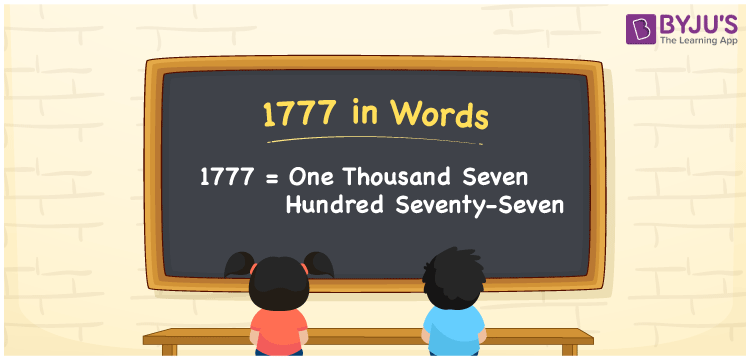# 1777 in Words

1777 in words is written as One thousand seven hundred seventy-seven. In both the International System of Numerals and the Indian System of Numerals, 1777 is written as One thousand seven hundred seventy-seven. The number 1777 is a Cardinal Number as it represents some quantity. For example, “the train tickets cost 1777 rupees”.

 1777 in Words One thousand seven hundred seventy-seven One thousand seven hundred seventy-seven in Number 1777

## 1777 in English Words

1777 in English words is read as “One thousand seven hundred seventy-seven.”## How to Write 1777 in Words?

To write 1777 in words, we shall use the place value chart. In the place value chart, put 1 in the thousands, 7 in the hundreds, tens and ones, respectively. Let us make a place value chart to write the number 1777 in words.

 Thousands Hundreds Tens Ones 1 7 7 7

Thus, we can write the expanded form as

1 × Thousand + 7 × Hundred + 7 × Ten + 7 × One

= 1 × 1000 + 7 × 100 + 7 × 10 + 7 × 1

= 1000 + 700 + 70 + 7

= 1777

= One thousand seven hundred seventy-seven.

1777 is the natural number that is succeeded by 1776 and preceded by 1778.

1777 in words – One thousand seven hundred seventy-seven

• Is 1777 an odd number? – Yes
• Is 1777 an even number? – No
• Is 1777 a perfect square number? – No
• Is 1777 a perfect cube number? – No
• Is 1777 a prime number? – Yes
• Is 1777 a composite number? – No

## Frequently Asked Questions on 1777 in Words

Q1

### How to write 1777 in words?

1777 in words is written as One thousand seven hundred seventy-seven.
Q2

### How to write 1777 in words in the International and Indian System of Numerals?

In both, the system of numerals, 1777 in words, is written as One thousand seven hundred seventy-seven.
Q3

### Is 1777 a prime number?

Yes, 1777 is the 275th prime number.